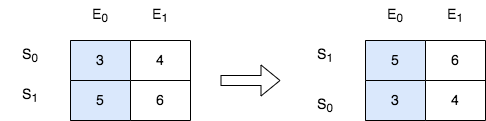2545. Sort the Students by Their Kth Score

#### QUESTION:

There is a class with m students and n exams. You are given a 0-indexed m x n integer matrix score, where each row represents one student and score[i][j] denotes the score the ith student got in the jth exam. The matrix score contains distinct integers only.

You are also given an integer k. Sort the students (i.e., the rows of the matrix) by their scores in the kth (0-indexed) exam from the highest to the lowest.

Return the matrix after sorting it.

Example 1:``````Input: score = [[10,6,9,1],[7,5,11,2],[4,8,3,15]], k = 2
Output: [[7,5,11,2],[10,6,9,1],[4,8,3,15]]
Explanation: In the above diagram, S denotes the student, while E denotes the exam.
- The student with index 1 scored 11 in exam 2, which is the highest score, so they got first place.
- The student with index 0 scored 9 in exam 2, which is the second highest score, so they got second place.
- The student with index 2 scored 3 in exam 2, which is the lowest score, so they got third place.
``````

Example 2:``````Input: score = [[3,4],[5,6]], k = 0
Output: [[5,6],[3,4]]
Explanation: In the above diagram, S denotes the student, while E denotes the exam.
- The student with index 1 scored 5 in exam 0, which is the highest score, so they got first place.
- The student with index 0 scored 3 in exam 0, which is the lowest score, so they got second place.
``````

Constraints:

``````m == score.length
n == score[i].length
1 <= m, n <= 250
1 <= score[i][j] <= 105
score consists of distinct integers.
0 <= k < n
``````

#### EXPLANATION:

easy的题目, 直接进行排序即可.

#### SOLUTION:

``````class Solution {
func sortTheStudents(_ score: [[Int]], _ k: Int) -> [[Int]] {
var score = score
var sorted = score.sorted { a, b in
return a[k] > b[k]
}
return sorted
}
}
``````